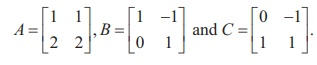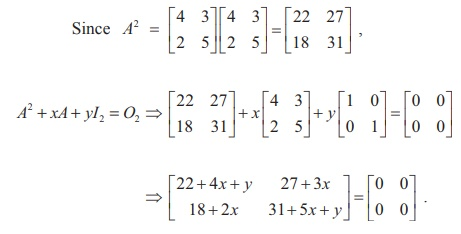Home | | Maths 12th Std | Properties of inverses of matrices

# Properties of inverses of matrices

We state and prove some theorems on non-singular matrices.

Properties of inverses of matrices

We state and prove some theorems on non-singular matrices.

### Theorem 1.4

If A is non-singular, then, where ╬╗ is a non-zero scalar.

### Proof

Let A be non-singular. Then |A| Ôëá 0 and AÔłĺ1 exists. By definition,### Theorem 1.5 (Left Cancellation Law)

Let A, B, and C be square matrices of order n. If A is non-singular and AB = AC, then B = C.

Proof

Since A is non-singular, AÔłĺ1 exists and AAÔłĺ1 = AÔłĺ1 A = In . Taking AB = AC and pre-multiplying both sides by AÔłĺ1, we get AÔłĺ1 ( AB) = AÔłĺ1 ( AC). By using the associative property of matrix multiplication and property of inverse matrix, we get B = C.

### Theorem1.6 (Right Cancellation Law)

Let A, B, and C be square matrices of order n. If A is non-singular and BA = CA, then B = C.

Proof

Since A is non-singular, AÔłĺ1 exists and AAÔłĺ1 = AÔłĺ1 A = In . Taking BA = CA and post-multiplying both sides by AÔłĺ1, we get (BA) AÔłĺ1 = (CA) AÔłĺ1. By using the associative property of matrix multiplication and property of inverse matrix, we get B = C.

Note

If A is singular and AB = AC or BA = CA, then B and C need not be equal. For instance, consider the following matrices:We note that |A| = 0 and AB = AC ; . but B  Ôëá C

Theorem 1.7 (Reversal Law for Inverses)

If A and B are non-singular matrices of the same order, then the product AB is also non-singular and ( AB)Ôłĺ1 = BÔłĺ1 AÔłĺ1.

Proof

Assume that A and B are non-singular matrices of same order n. Then,| A | Ôëá 0, | B | Ôëá 0, both AÔłĺ1 and BÔłĺ1 exist and they are of order n. The products AB and BÔłĺ1 AÔłĺ1 can be found and they are also of order n. Using the product rule for determinants, we get |AB| =| A || B |Ôëá 0. So, AB is non-singular and

( AB)(B-1 A-1 ) = ( A(BB-1 )) A-1 = ( AIn ) A-1 = AA-1 = In ;

(B-1 A-1 )( AB) = (B-1 ( A-1 A))B = (B-1In )B = B-1B = In .

Hence ( AB)Ôłĺ1 = BÔłĺ1 AÔłĺ1.

Theorem 1.8 (Law of Double Inverse)

If A is non-singular, then AÔłĺ1 is also non-singular and ( AÔłĺ1 )Ôłĺ1 = A.

Proof

Assume that A is non-singular. Then |A | Ôëá 0, and AÔłĺ1 exists.

Now, |A-1| = 1/|A| Ôëá 0

Ôçĺ  A-1 is also non-singular, and AA-1 = A-1A = I

Now, A-1A = I  Ôçĺ (AA-1)-1 = I Ôçĺ (A-1)-1 A-1 = I.

Post-multiplying by A on both sides of equation (1), we get (A-1)-1 =A.

### Theorem 1.9

If A is a non-singular square matrix of order n , thenProof

Since A is a non-singular square matrix, we have |A| Ôëá 0 and so, we getNote

If A is a non-singular matrix of order 3, then, |A | Ôëá 0 . By theorem 1.9 (ii), we get |adjA| = | A|2 and so, | adj A | is positive. Then, we get |A| = ┬▒ ÔłÜ|adjA| .

So, we getFurther, by property (iii), we getHence, if A is a non-singular matrix of order 3, then we getExample 1.4

If A is a non-singular matrix of odd order, prove that |adj A| is positive.

Solution

Let A be a non-singular matrix of order 2m + 1 , where m = 0,1, 2, .. . . Then, we get |A| Ôëá 0 and, by theorem 1.9 (ii), we have |adj A| = |A|(2m+1)-1 = |A|2m

Since |A|2m is always positive, we get that |adj A| is positive.

Example 1.5

Find a matrix  A if adj( A)SolutionExample 1.6

If adj A =find AÔłĺ1.

SolutionExample1.7

If A is symmetric, prove that adj A is also symmetric.

Solution

Suppose A is symmetric. Then, AT = A and so, by theorem 1.9 (vi), we get

Theorem 1.10

If A and B are any two non-singular square matrices of order n , then

Proof

Replacing A by AB in adj(A) = |A|AÔłĺ1 , we get

### Example 1.8

Verify the property  ( AT  )Ôłĺ1  = ( AÔłĺ1 )T  with  A.

### Solution

For the given A, we get |A |= (2) (7) -  (9)(1) = 14 Ôłĺ 9 = 5 .From (1) and (2), we get (A-1) = (AT)-1. Thus, we have verified the given property.

Example 1.9

Verify ( AB)Ôłĺ1 = BÔłĺ1 AÔłĺ1 withSolutionAs the matrices in (1) and (2) are same, (AB) Ôłĺ1 = B-1 A-1 is verified.

### Example 1.10

If  A  =, find x and y such that A2 + xA + yI2=O2, Hence, find AÔłĺ1.

SolutionSo, we get 22 + 4x + y =0, 31+5x+y=0, 27+3x=0 and 18+2x=0

Hence x = Ôłĺ9 and y =14.Then, we get A2 - 9A + 14I2 = O2

Post-multiplying this equation by AÔłĺ1 , we get A ÔÇô 9I2 + 14A-1 = O2.  Hence, we getTags : Definition, Theorem, Formulas, Solved Example Problems | Inverse of a Non-Singular Square Matrix , 12th Mathematics : UNIT 1 : Applications of Matrices and Determinants
Study Material, Lecturing Notes, Assignment, Reference, Wiki description explanation, brief detail
12th Mathematics : UNIT 1 : Applications of Matrices and Determinants : Properties of inverses of matrices | Definition, Theorem, Formulas, Solved Example Problems | Inverse of a Non-Singular Square Matrix